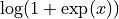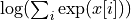# Aesara at a Glance¶

Aesara is a Python library that lets you define, optimize, and evaluate mathematical expressions, especially ones involving multi-dimensional arrays (e.g. numpy.ndarrays). Using Aesara it is possible to attain speeds rivaling hand-crafted C implementations for problems involving large amounts of data.

Aesara combines aspects of a computer algebra system (CAS) with aspects of an optimizing compiler. It can also generate customized C code for many mathematical operations. This combination of CAS with optimizing compilation is particularly useful for tasks in which complicated mathematical expressions are evaluated repeatedly and evaluation speed is critical. For situations where many different expressions are each evaluated once, Aesara can minimize the amount of compilation/analysis overhead, but still provide symbolic features such as automatic differentiation.

Aesara’s compiler applies many optimizations of varying complexity to these symbolic expressions. These optimizations include, but are not limited to:

• constant folding
• merging of similar subgraphs, to avoid redundant calculation
• arithmetic simplification (e.g. x*y/x -> y, --x -> x)
• inserting efficient BLAS operations (e.g. GEMM) in a variety of contexts
• using memory aliasing to avoid unnecessary calculations
• using in-place operations wherever it does not interfere with aliasing
• loop fusion for element-wise sub-expressions
• improvements to numerical stability (e.g.and)

The library that Aesara is based on, Theano, was written at the LISA lab to support rapid development of efficient machine learning algorithms. Theano was named after the Greek mathematician, who may have been Pythagoras’ wife. Aesara is an alleged daughter of Pythagoras and Theano.

## Sneak peek¶

Here is an example of how to use Aesara. It doesn’t show off many of its features, but it illustrates concretely what Aesara is.

import aesara
from aesara import tensor as aet

# declare two symbolic floating-point scalars
a = aet.dscalar()
b = aet.dscalar()

# create a simple expression
c = a + b

# convert the expression into a callable object that takes (a, b)
# values as input and computes a value for c
f = aesara.function([a, b], c)

# bind 1.5 to 'a', 2.5 to 'b', and evaluate 'c'
assert 4.0 == f(1.5, 2.5)


Aesara is not a programming language in the normal sense because you write a program in Python that builds expressions for Aesara. Still it is like a programming language in the sense that you have to

• declare variables a and b and give their types,
• build expressions graphs using those variables,
• compile the expression graphs into functions that can be used for computation.

It is good to think of aesara.function() as the interface to a compiler which builds a callable object from a purely symbolic graph. One of Aesara’s most important features is that aesara.function() can optimize a graph and even compile some or all of it into native machine instructions.

## What does it do that NumPy doesn’t¶

Aesara is a essentially an optimizing compiler for manipulating and evaluating expressions, especially tensor-valued ones. Manipulation of tensors is typically done using the NumPy package, so what does Aesara do that Python and NumPy don’t do?

• execution speed optimizations: Aesara can use C, Numba, or JAX to compile parts your expression graph into CPU or GPU instructions, which run much faster than pure Python.
• symbolic differentiation: Aesara can automatically build symbolic graphs for computing gradients.
• stability optimizations: Aesara can recognize some numerically unstable expressions and compute them with more stable algorithms.

The closest Python package to Aesara is sympy. Aesara focuses more on tensor expressions than Sympy, and has more machinery for compilation. Sympy has more sophisticated algebra rules and can handle a wider variety of mathematical operations (such as series, limits, and integrals).

If numpy is to be compared to MATLAB and sympy to Mathematica, Aesara is a sort of hybrid of the two which tries to combine the best of both worlds.

## Getting started¶

Installing Aesara